# NCERT Class 8 Maths Chapter 7 – Cubes and Cube roots solutions

### Access Answers of Maths NCERT Class 8 Chapter 7 – Cubes and Cube roots

Exercise 7.1 Page: 114

1. Which of the following numbers are not perfect cubes?

(i) 216

Solution:

By resolving 216 into prime factor,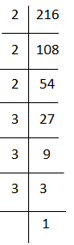216 = 2×2×2×3×3×3

By grouping the factors in triplets of equal factors, 216 = (2×2×2)×(3×3×3)

Here, 216 can be grouped into triplets of equal factors,

∴ 216 = (2×3) = 6

Hence, 216 is cube of 6.

(ii) 128

Solution:

By resolving 128 into prime factor,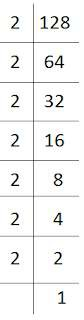128 = 2×2×2×2×2×2×2

By grouping the factors in triplets of equal factors, 128 = (2×2×2)×(2×2×2)×2

Here, 128 cannot be grouped into triplets of equal factors, we are left of with one factors 2 .

∴ 128 is not a perfect cube.

(iii) 1000 Solution:

By resolving 1000 into prime factor,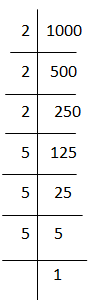1000 = 2×2×2×5×5×5

By grouping the factors in triplets of equal factors, 1000 = (2×2×2)×(5×5×5)

Here, 1000 can be grouped into triplets of equal factors,

∴ 1000 = (2×5) = 10

Hence, 1000 is cube of 10.

(iv) 100 Solution:

By resolving 100 into prime factor,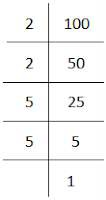100 = 2×2×5×5

Here, 100 cannot be grouped into triplets of equal factors.

∴ 100 is not a perfect cube.

(v) 46656

Solution:

By resolving 46656 into prime factor,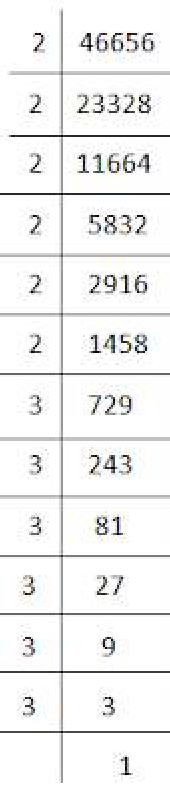46656 = 2×2×2×2×2×2×3×3×3×3×3×3

By grouping the factors in triplets of equal factors, 46656 = (2×2×2)×(2×2×2)×(3×3×3)×(3×3×3)

Here, 46656 can be grouped into triplets of equal factors,

∴ 46656 = (2×2×3×3) = 36

Hence, 46656 is cube of 36.

2. Find the smallest number by which each of the following numbers must be multiplied to obtain a perfect cube.

(i) 243

Solution:

By resolving 243 into prime factor,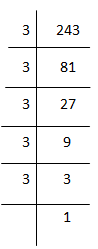243 = 3×3×3×3×3

By grouping the factors in triplets of equal factors, 243 = (3×3×3)×3×3

Here, 3 cannot be grouped into triplets of equal factors.

∴ We will multiply 243 by 3 to get perfect cube.

(ii) 256 Solution:

By resolving 256 into prime factor,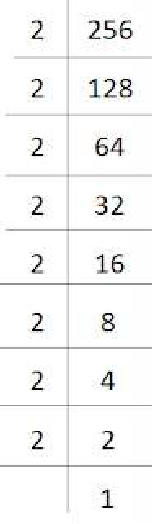256 = 2×2×2×2×2×2×2×2

By grouping the factors in triplets of equal factors, 256 = (2×2×2)×(2×2×2)×2×2

Here, 2 cannot be grouped into triplets of equal factors.

∴ We will multiply 256 by 2 to get perfect cube.

(iii) 72

Solution:

By resolving 72 into prime factor,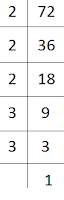72 = 2×2×2×3×3

By grouping the factors in triplets of equal factors, 72 = (2×2×2)×3×3

Here, 3 cannot be grouped into triplets of equal factors.

∴ We will multiply 72 by 3 to get perfect cube.

(iv) 675 Solution:

By resolving 675 into prime factor,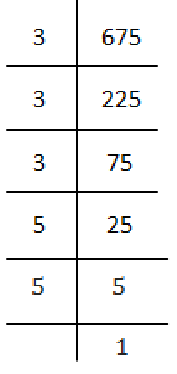675 = 3×3×3×5×5

By grouping the factors in triplets of equal factors, 675 = (3×3×3)×5×5

Here, 5 cannot be grouped into triplets of equal factors.

∴ We will multiply 675 by 5 to get perfect cube.

(v) 100 Solution:

By resolving 100 into prime factor,100 = 2×2×5×5

Here, 2 and 5 cannot be grouped into triplets of equal factors.

∴ We will multiply 100 by (2×5) 10 to get perfect cube.

3. Find the smallest number by which each of the following numbers must be divided to obtain a perfect cube.

(i) 81

Solution:

By resolving 81 into prime factor,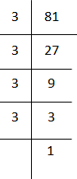81 = 3×3×3×3

By grouping the factors in triplets of equal factors, 81 = (3×3×3)×3

Here, 3 cannot be grouped into triplets of equal factors.

∴ We will divide 81 by 3 to get perfect cube.

(ii) 128 Solution:

By resolving 128 into prime factor,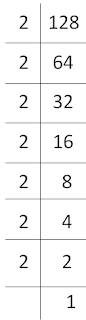128 = 2×2×2×2×2×2×2

By grouping the factors in triplets of equal factors, 128 = (2×2×2)×(2×2×2)×2

Here, 2 cannot be grouped into triplets of equal factors.

∴ We will divide 128 by 2 to get perfect cube.

(iii) 135 Solution:

By resolving 135 into prime factor,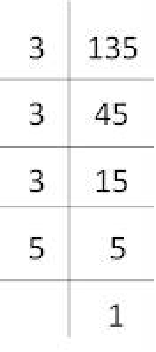135 = 3×3×3×5

By grouping the factors in triplets of equal factors, 135 = (3×3×3)×5

Here, 5 cannot be grouped into triplets of equal factors.

∴ We will divide 135 by 5 to get perfect cube.

(iv) 192 Solution:

By resolving 192 into prime factor,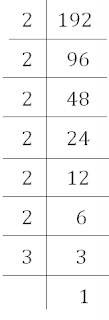192 = 2×2×2×2×2×2×3

By grouping the factors in triplets of equal factors, 192 = (2×2×2)×(2×2×2)×3

Here, 3 cannot be grouped into triplets of equal factors.

∴ We will divide 192 by 3 to get perfect cube.

(v) 704 Solution:

By resolving 704 into prime factor,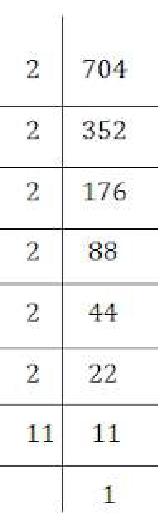704 = 2×2×2×2×2×2×11

By grouping the factors in triplets of equal factors, 704 = (2×2×2)×(2×2×2)×11

Here, 11 cannot be grouped into triplets of equal factors.

∴ We will divide 704 by 11 to get perfect cube.

4. Parikshit makes a cuboid of plasticine of sides 5 cm, 2 cm, 5 cm. How many such cuboids will he need to form a cube?

Solution:

Given, side of cube is 5 cm, 2 cm and 5 cm.

∴ Volume of cube = 5×2×5 = 50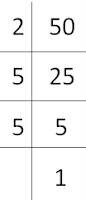50 = 2×5×5

Here, 2 , 5 and 5 cannot be grouped into triplets of equal factors.

∴ We will multiply 50 by (2×2×5) 20 to get perfect cube. Hence, 20 cuboid is needed.

## Exercise 7.2 Page: 116

1. Find the cube root of each of the following numbers by prime factorisation method.

(i) 64

Solution:

64 = 2×2×2×2×2×2

By grouping the factors in triplets of equal factors, 64 = (2×2×2)×(2×2×2)

Here, 64 can be grouped into triplets of equal factors,

∴ 64 = 2×2 = 4

Hence, 4 is cube root of 64.

(ii) 512

Solution:

512 = 2×2×2×2×2×2×2×2×2

By grouping the factors in triplets of equal factors, 512 = (2×2×2)×(2×2×2)×(2×2×2)

Here, 512 can be grouped into triplets of equal factors,

∴ 512 = 2×2×2 = 8

Hence, 8 is cube root of 512.

(iii) 10648

Solution:

10648 = 2×2×2×11×11×11

By grouping the factors in triplets of equal factors, 10648 = (2×2×2)×(11×11×11)

Here, 10648 can be grouped into triplets of equal factors,

∴ 10648 = 2 ×11 = 22

Hence, 22 is cube root of 10648.

(iv) 27000

Solution:

27000 = 2×2×2×3×3×3×3×5×5×5

By grouping the factors in triplets of equal factors, 27000 = (2×2×2)×(3×3×3)×(5×5×5)

Here, 27000 can be grouped into triplets of equal factors,

∴ 27000 = (2×3×5) = 30

Hence, 30 is cube root of 27000.

(v) 15625

Solution:

15625 = 5×5×5×5×5×5

By grouping the factors in triplets of equal factors, 15625 = (5×5×5)×(5×5×5)

Here, 15625 can be grouped into triplets of equal factors,

∴ 15625 = (5×5) = 25

Hence, 25 is cube root of 15625.

(vi) 13824

Solution:

13824 = 2×2×2×2×2×2×2×2×2×3×3×3

By grouping the factors in triplets of equal factors,

13824 = (2×2×2)×(2×2×2)×(2×2×2)×(3×3×3)

Here, 13824 can be grouped into triplets of equal factors,

∴ 13824 = (2×2× 2×3) = 24

Hence, 24 is cube root of 13824.

(vii) 110592

Solution:

110592 = 2×2×2×2×2×2×2×2×2×2×2×2×3×3×3

By grouping the factors in triplets of equal factors,

110592 = (2×2×2)×(2×2×2)×(2×2×2)×(2×2×2)×(3×3×3)

Here, 110592 can be grouped into triplets of equal factors,

∴ 110592 = (2×2×2×2 × 3) = 48

Hence, 48 is cube root of 110592.

(viii) 46656

Solution:

46656 = 2×2×2×2×2×2×3×3×3×3×3×3

By grouping the factors in triplets of equal factors,

46656 = (2×2×2)×(2×2×2)×(3×3×3)×(3×3×3)

Here, 46656 can be grouped into triplets of equal factors,

∴ 46656 = (2×2×3×3) = 36

Hence, 36 is cube root of 46656.

(ix) 175616

Solution:

175616 = 2×2×2×2×2×2×2×2×2×7×7×7

By grouping the factors in triplets of equal factors,

175616 = (2×2×2)×(2×2×2)×(2×2×2)×(7×7×7)

Here, 175616 can be grouped into triplets of equal factors,

∴ 175616 = (2×2×2×7) = 56

Hence, 56 is cube root of 175616.

(x) 91125

Solution:

91125 = 3×3×3×3×3×3×3×5×5×5

By grouping the factors in triplets of equal factors, 91125 = (3×3×3)×(3×3×3)×(5×5×5)

Here, 91125 can be grouped into triplets of equal factors,

∴ 91125 = (3×3×5) = 45

Hence, 45 is cube root of 91125.

2. State true or false.

(i) Cube of any odd number is even.

Solution:

False

(ii) A perfect cube does not end with two zeros.

Solution:

True

(iii) If cube of a number ends with 5, then its cube ends with 25.

Solution:

False

(iv) There is no perfect cube which ends with 8.

Solution:

False

(v) The cube of a two digit number may be a three digit number.

Solution:

False

(vi) The cube of a two digit number may have seven or more digits.

Solution:

False

(vii) The cube of a single digit number may be a single digit number.

Solution:

True

3. You are told that 1,331 is a perfect cube. Can you guess without factorisation what is its cube root? Similarly, guess the cube roots of 4913, 12167, 32768.

Solution:

(i) By grouping the digits, we get 1 and 331

We know that, since, the unit digit of cube is 1, the unit digit of cube root is 1.

∴ We get 1 as unit digit of the cube root of 1331.

The cube of 1 matches with the number of second group.

∴ The ten’s digit of our cube root is taken as the unit place of smallest number.

We know that, the unit’s digit of the cube of a number having digit as unit’s place 1 is 1.

∴ ∛1331 = 11

(ii) By grouping the digits, we get 4 and 913

We know that, since, the unit digit of cube is 3, the unit digit of cube root is 7.

∴ we get 7 as unit digit of the cube root of 4913. We know 13 = 1 and 23 = 8 , 1 > 4 > 8.

Thus, 1 is taken as ten digit of cube root.

∴ ∛4913 = 17

(iii) By grouping the digits, we get 12 and 167.

We know that, since, the unit digit of cube is 7, the unit digit of cube root is 3.

∴ 3 is the unit digit of the cube root of 12167 We know 23 = 8 and 33 = 27 , 8 > 12 > 27.

Thus, 2 is taken as ten digit of cube root.

∴ ∛12167= 23

(iv) By grouping the digits, we get 32 and 768.

We know that, since, the unit digit of cube is 8, the unit digit of cube root is 2.

∴ 2 is the unit digit of the cube root of 32768. We know 33 = 27 and 43 = 64 , 27 > 32 > 64.

Thus, 3 is taken as ten digit of cube root.

∴ ∛32768= 32